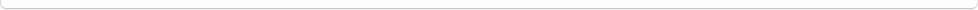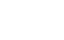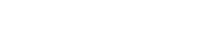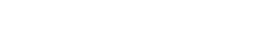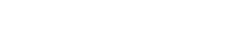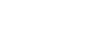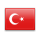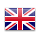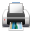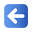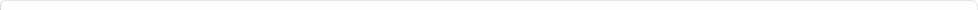Description of Individual Course Units
 Course Unit Code Course Unit Title Type of Course Unit Year of Study Semester Number of ECTS Credits 140400103105 STATISTICS-I Compulsory 2 3 4
Level of Course Unit
First Cycle
Objectives of the Course
This course aims to introduce the main concepts of statistics to the student, have them basic knowledge and skills required for gathering, organizing, analyzing and interpreting data for research.
Name of Lecturer(s)
Learning Outcomes
 1 Define basic statistical concepts. 2 Organize business data using appropriate statistical tools (statistical series, graphs and tables). 3 Summarize and interpret statistical series by choosing most appropriate descriptive statistical measures. 4 Express basic probability concepts and rules and apply probability rules to real case problems accurately. 5 Choose most appropriate probability distribution among from most widely used probability distributions and apply them to real cases.
Mode of Delivery
Daytime Class
Prerequisites and co-requisities
None
Recommended Optional Programme Components
None
Course Contents
Definition of Statistics and basic concepts, data types and methods of data collection, arrangement and presentation of data, measures of central tendency, Measures of Dispersion, Probability theory and random variables, estimation theory
Weekly Detailed Course Contents
 Week Theoretical Practice Laboratory 1 Basic statistical concepts 2 Frequency Distributions, Histograms and Frequency Ranges 3 Measures of parametric central tendency 4 Measures of nonparametric central tendency 5 Measures of Parametric Dispersion 6 Measures of Nonparametric Dispersion 7 Moments, Skewness and Kurtosis Coefficients 8 Probability Theory, Basic Concepts 9 Bayes' Theorem, Probability Distribution Table, Expected Value 10 Discrete Probability Distributions 11 Binomial Distribution, Poisson Distribution 12 Hypergeometric distribution 13 Normal Distribution, Standard Normal Curve Areas 14 15 16
Planned Learning Activities and Teaching Methods
Assessment Methods and Criteria
 Term (or Year) Learning Activities Quantity Weight Midterm Examination 1 100 SUM 100 End Of Term (or Year) Learning Activities Quantity Weight Final Examination 1 100 SUM 100 Term (or Year) Learning Activities 40 End Of Term (or Year) Learning Activities 60 SUM 100
Language of Instruction
Turkish
Work Placement(s)
None